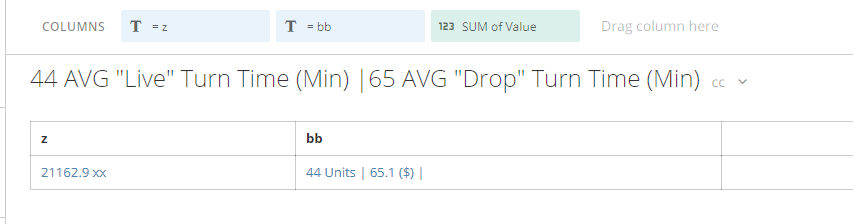# Beast Mode works but not in Summary

I have created a beastmode calculation to show as my summary. The calculation works, however when I select it to show as a summary number it returns "No Summary Number".

My beast mode is:

CONCAT(ROUND(AVG(CASE
when LOWER(`On-Time or Late`) = 'on-time' and LOWER(`Drop/Live`) = 'live' then ABS(`Live Turn Time Data`)
when LOWER(`On-Time or Late`) = 'late' and LOWER(`Drop/Live`) = 'live' then ABS(`Live Late Turn Time Data`)
END)), ' AVG "Live" Turn Time (Min) |',

ROUND(AVG(CASE
when LOWER(`On-Time or Late`) = 'on-time' and LOWER(`Drop/Live`) = 'drop' then ABS(`Drop Turn Time Data`)
when LOWER(`On-Time or Late`) = 'late' and LOWER(`Drop/Live`) = 'drop' then ABS(`Drop Late Turn Time Data`)
END)), ' AVG "Drop" Turn Time (Min)')

• Using my own dataset, I can get the beast calc to show in the summary value:

The difference is I moved the ABS to the front. Hope that helps

CONCAT(
round(abs(avg(case when (`Account Name`) = 'Units' then `Value` end )),0) , ' Units | ' ,
round(abs(avg(case when (`Account Name`) = 'Price' then `Value` end )),2) , ' (\$) |'
)

In your case, try the below .. let me know if that works

CONCAT(
ROUND(abs(AVG(CASE
when LOWER(`On-Time or Late`) = 'on-time' and LOWER(`Drop/Live`) = 'live' then (`Live Turn Time Data`)
when LOWER(`On-Time or Late`) = 'late' and LOWER(`Drop/Live`) = 'live' then (`Live Late Turn Time Data
END))), ' AVG "Live" Turn Time (Min) |',
ROUND(abs(AVG(CASE
when LOWER(`On-Time or Late`) = 'on-time' and LOWER(`Drop/Live`) = 'drop' then (`Drop Turn Time Data`)
when LOWER(`On-Time or Late`) = 'late' and LOWER(`Drop/Live`) = 'drop' then (`Drop Late Turn Time Data`)END))), ' AVG "Drop" Turn Time (Min)')• Save the card as is with the BM in there,  Close your browser and try again,  The code looks ok and contains an aggregation..and if it runs in like a table or something it should work on sum num,

• Thank you for the input. I did close the browser and re-try, but no luck.

I should clarify something on my best mode. The formula does not seem to contain any syntax errors and I have validated the calculation within the beast mode itself. It does not, however, return any data when the card is in table form.

Any suggestions are greatly appreciated.

• Using my own dataset, I can get the beast calc to show in the summary value:

The difference is I moved the ABS to the front. Hope that helps

CONCAT(
round(abs(avg(case when (`Account Name`) = 'Units' then `Value` end )),0) , ' Units | ' ,
round(abs(avg(case when (`Account Name`) = 'Price' then `Value` end )),2) , ' (\$) |'
)

In your case, try the below .. let me know if that works

CONCAT(
ROUND(abs(AVG(CASE
when LOWER(`On-Time or Late`) = 'on-time' and LOWER(`Drop/Live`) = 'live' then (`Live Turn Time Data`)
when LOWER(`On-Time or Late`) = 'late' and LOWER(`Drop/Live`) = 'live' then (`Live Late Turn Time Data
END))), ' AVG "Live" Turn Time (Min) |',
ROUND(abs(AVG(CASE
when LOWER(`On-Time or Late`) = 'on-time' and LOWER(`Drop/Live`) = 'drop' then (`Drop Turn Time Data`)
when LOWER(`On-Time or Late`) = 'late' and LOWER(`Drop/Live`) = 'drop' then (`Drop Late Turn Time Data`)END))), ' AVG "Drop" Turn Time (Min)')• Thank you so very much Tadashii!

• In hindsight, the ABS should be placed after AVG so that you can average the absolute numbers correctly  ?

ROUND(AVG(ABS(CASE